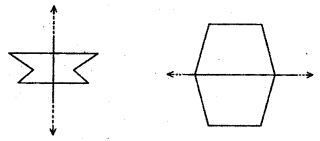# Class 6 Maths NCERT Solutions for Chapter 13 Symmetry Ex – 13.2

## Symmetry

Question 1.
Find the number of lines of symmetry for each of the following shapes.Solution:
Each one of the given figures are symmetrical about the dotted line(s) drawn. The number lines of symmetry are indicated against each figure: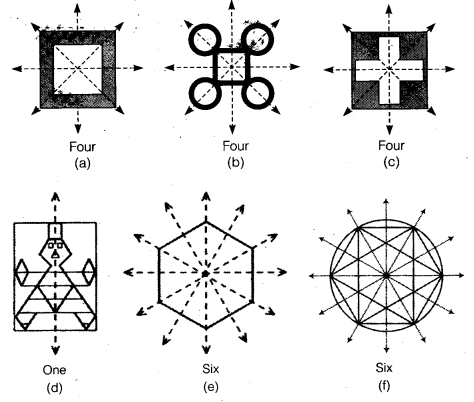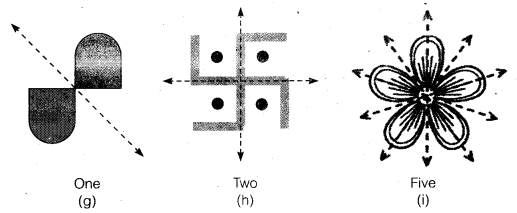Question 2.
Copy the triangle in each of the following figures on squared paper. In each case, draw the line(s) of symmetry, if any and identify the type of triangle. (Some of you may like to trace the figures and try paper-folding first!)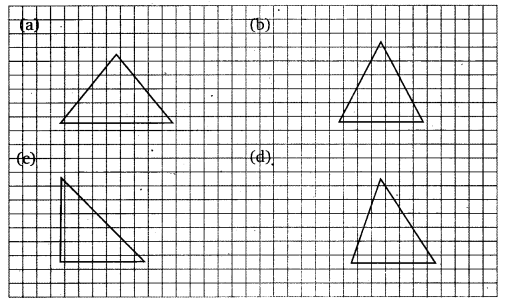Solution:
(a), (b) and (d) are isosceles triangles, (c) is a right angled isosceles triangle. Their line(s) of symmetry marked with dotted line(s) in each case as under: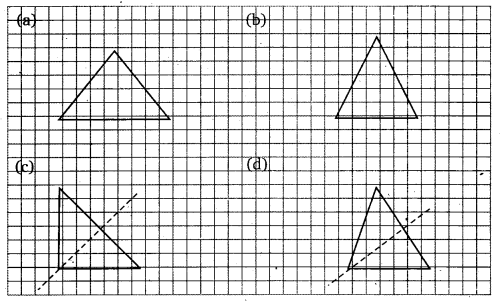Question 3.
Complete the following table :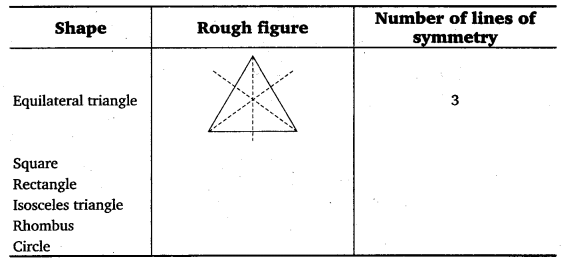Solution:
Complete table with rough figures duly filled is as under: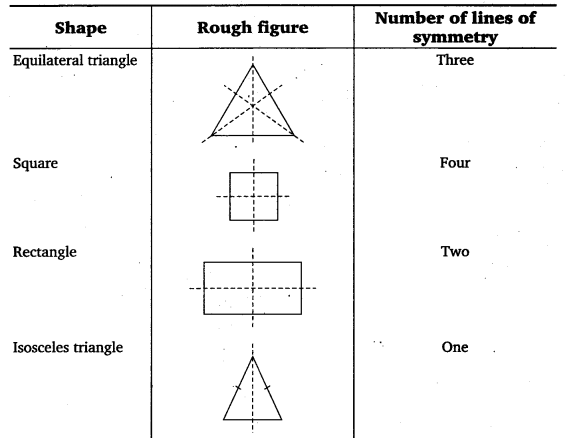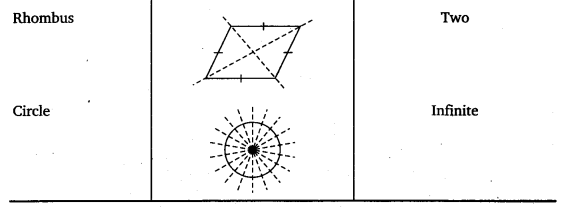Question 4.
Can you draw a triangle which has

(a) exactly one. line of symmetry?
(b) exactly two lines of symmetry?
(c) exactly three lines of symmetry?
(d) no lines of symmetry? Sketch a rough figure in each case.

Solution:
(a) Yes, it is an isosceles triangle. Its rough sketch is as shown.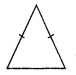(b) No.
(c) Yes, it is an equilateral triangle. Its rough sketch is as shown.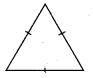(d) Yes, it is a scalene triangle. Its rough sketch is as shown.Question 5.
On a squared paper, sketch the following:

(a) A triangle with a horizontal line of symmetry but no vertical line of symmetry.
(b) A quadrilateral with both horizontal and vertical lines of symmetry.
(c) A quadrilateral with a horizontal line of symmetry but no vertical line of symmetry.
(d) A hexagon with exactly two lines of symmetry.
(e) A hexagon with six lines of symmetry.

Solution:
Sketches of the required figure with their line(s) of symmetry are shown as under: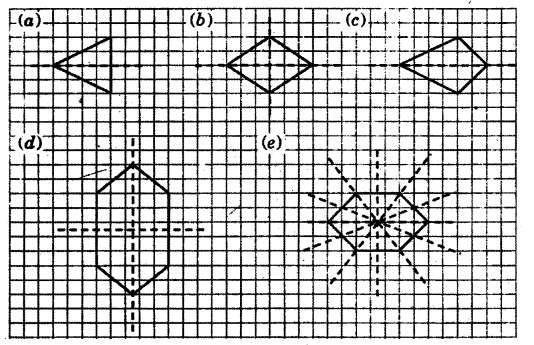Question 6.
Trace each figure and draw the lines of symmetry, if any:Solution:
The line(s) of symmetry of the given figures are shown as dotted lines as under: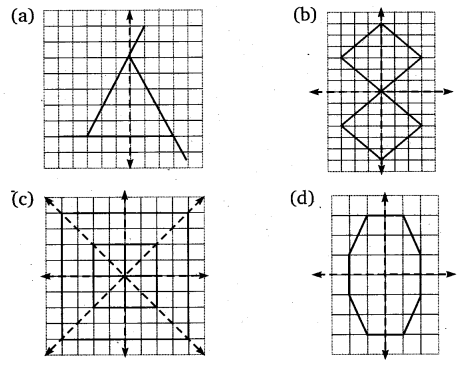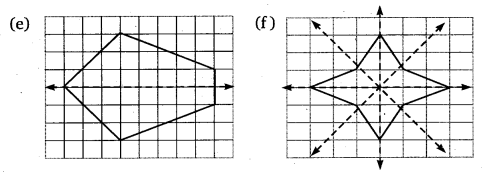Question 7.
Consider the letters of English alphabets, A to Z.

List among them the letters which have

(a) vertical lines of symmetry (like A)
(b) horizontal lines of symmetry (like B)
(c) no lines of symmetry (like Q)

Solution:
The English alphabets A to Z having
(a) vertical lines of symmetry (like A) are
A, H, I, M, O, T, U, V, W, X and Y.
(b) horizontal lines of symmetry (like B) are
B, C, D, E, H, I, K, O and X.
(c) no lines of symmetry (like Q) are G, J, L, P, Q, R, S and Z.

Question 8.
Given here are figures of a few folded sheets and designs drawn about the fold. In each case, draw a rough diagram of the complete figure that would be seen when the design is cut off.Solution:
The rough diagram of the complete figure that would be seen when the design is cut off is as under: